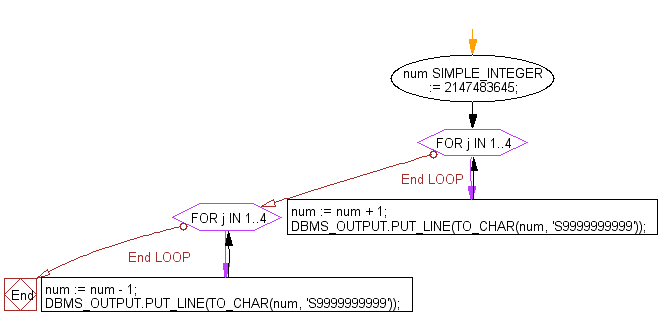﻿ PL/SQL DataType Exercises: Program to show the uses of SIMPLE_INTEGER datatype - w3resource# PL/SQL DataType: Program to show the uses of SIMPLE_INTEGER datatype

## PL/SQL DataType: Exercise-6 with Solution

Write a PL/SQL program to show the uses of SIMPLE_INTEGER datatype.

Sample Solution:

PL/SQL Code:

``````DECLARE
num SIMPLE_INTEGER := 2147483645;
BEGIN
FOR j IN 1..4 LOOP
num := num + 1;
DBMS_OUTPUT.PUT_LINE(TO_CHAR(num, 'S9999999999'));
END LOOP;
FOR j IN 1..4 LOOP
num := num - 1;
DBMS_OUTPUT.PUT_LINE(TO_CHAR(num, 'S9999999999'));
END LOOP;
END;
/
```
```

Flowchart:Sample Output:

```+2147483646
+2147483647
-2147483648
-2147483647
-2147483648
+2147483647
+2147483646
+2147483645

PL/SQL procedure successfully completed.
```

In the above example the initial value is 2147483645, when this value reach in upper limit it started from starting value.

Improve this sample solution and post your code through Disqus

What is the difficulty level of this exercise?

﻿